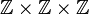Free abelian group of rank three

The free abelian group of rank three is defined as the free abelian group of rank three. Explicitly, it is defined as the external direct product of three copies of the group of integers, and is denoted$\mathbb{Z} \times \mathbb{Z} \times \mathbb{Z}$ or$\mathbb{Z}^3$.